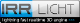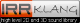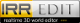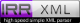Retour
Version Originale

#### ./aip/1.8aipmod/include/vector2d.h :


// Copyright (C) 2002-2011 Nikolaus Gebhardt

// This file is part of the "Irrlicht Engine".

// For conditions of distribution and use, see copyright notice in irrlicht.h

#ifndef __IRR_POINT_2D_H_INCLUDED__
#define __IRR_POINT_2D_H_INCLUDED__

#include "irrMath.h"
#include "dimension2d.h"

namespace irr
{
namespace core
{

//! 2d vector template class with lots of operators and methods.

/** As of Irrlicht 1.6, this class supercedes position2d, which should
be considered deprecated. */
template <class T>
class vector2d
{
public:
//! Default constructor (null vector)

vector2d() : X(0), Y(0) {}
//! Constructor with two different values

vector2d(T nx, T ny) : X(nx), Y(ny) {}
//! Constructor with the same value for both members

explicit vector2d(T n) : X(n), Y(n) {}
//! Copy constructor

vector2d(const vector2d<T>& other) : X(other.X), Y(other.Y) {}

vector2d(const dimension2d<T>& other) : X(other.Width), Y(other.Height) {}

// operators

vector2d<T> operator-() const { return vector2d<T>(-X, -Y); }

vector2d<T>& operator=(const vector2d<T>& other) { X = other.X; Y = other.Y; return *this; }

vector2d<T>& operator=(const dimension2d<T>& other) { X = other.Width; Y = other.Height; return *this; }

vector2d<T> operator+(const vector2d<T>& other) const { return vector2d<T>(X + other.X, Y + other.Y); }
vector2d<T> operator+(const dimension2d<T>& other) const { return vector2d<T>(X + other.Width, Y + other.Height); }
vector2d<T>& operator+=(const vector2d<T>& other) { X+=other.X; Y+=other.Y; return *this; }
vector2d<T> operator+(const T v) const { return vector2d<T>(X + v, Y + v); }
vector2d<T>& operator+=(const T v) { X+=v; Y+=v; return *this; }
vector2d<T>& operator+=(const dimension2d<T>& other) { X += other.Width; Y += other.Height; return *this;  }

vector2d<T> operator-(const vector2d<T>& other) const { return vector2d<T>(X - other.X, Y - other.Y); }
vector2d<T> operator-(const dimension2d<T>& other) const { return vector2d<T>(X - other.Width, Y - other.Height); }
vector2d<T>& operator-=(const vector2d<T>& other) { X-=other.X; Y-=other.Y; return *this; }
vector2d<T> operator-(const T v) const { return vector2d<T>(X - v, Y - v); }
vector2d<T>& operator-=(const T v) { X-=v; Y-=v; return *this; }
vector2d<T>& operator-=(const dimension2d<T>& other) { X -= other.Width; Y -= other.Height; return *this;  }

vector2d<T> operator*(const vector2d<T>& other) const { return vector2d<T>(X * other.X, Y * other.Y); }
vector2d<T>& operator*=(const vector2d<T>& other) { X*=other.X; Y*=other.Y; return *this; }
vector2d<T> operator*(const T v) const { return vector2d<T>(X * v, Y * v); }
vector2d<T>& operator*=(const T v) { X*=v; Y*=v; return *this; }

vector2d<T> operator/(const vector2d<T>& other) const { return vector2d<T>(X / other.X, Y / other.Y); }
vector2d<T>& operator/=(const vector2d<T>& other) { X/=other.X; Y/=other.Y; return *this; }
vector2d<T> operator/(const T v) const { return vector2d<T>(X / v, Y / v); }
vector2d<T>& operator/=(const T v) { X/=v; Y/=v; return *this; }

//! sort in order X, Y. Equality with rounding tolerance.

bool operator<=(const vector2d<T>&other) const
{
return 	(X<other.X || core::equals(X, other.X)) ||
(core::equals(X, other.X) && (Y<other.Y || core::equals(Y, other.Y)));
}

//! sort in order X, Y. Equality with rounding tolerance.

bool operator>=(const vector2d<T>&other) const
{
return 	(X>other.X || core::equals(X, other.X)) ||
(core::equals(X, other.X) && (Y>other.Y || core::equals(Y, other.Y)));
}

//! sort in order X, Y. Difference must be above rounding tolerance.

bool operator<(const vector2d<T>&other) const
{
return 	(X<other.X && !core::equals(X, other.X)) ||
(core::equals(X, other.X) && Y<other.Y && !core::equals(Y, other.Y));
}

//! sort in order X, Y. Difference must be above rounding tolerance.

bool operator>(const vector2d<T>&other) const
{
return 	(X>other.X && !core::equals(X, other.X)) ||
(core::equals(X, other.X) && Y>other.Y && !core::equals(Y, other.Y));
}

bool operator==(const vector2d<T>& other) const { return equals(other); }
bool operator!=(const vector2d<T>& other) const { return !equals(other); }

// functions

//! Checks if this vector equals the other one.

/** Takes floating point rounding errors into account.
\param other Vector to compare with.
\return True if the two vector are (almost) equal, else false. */
bool equals(const vector2d<T>& other) const
{
return core::equals(X, other.X) && core::equals(Y, other.Y);
}

vector2d<T>& set(T nx, T ny) {X=nx; Y=ny; return *this; }
vector2d<T>& set(const vector2d<T>& p) { X=p.X; Y=p.Y; return *this; }

//! Gets the length of the vector.

/** \return The length of the vector. */
T getLength() const { return core::squareroot( X*X + Y*Y ); }

//! Get the squared length of this vector

/** This is useful because it is much faster than getLength().
\return The squared length of the vector. */
T getLengthSQ() const { return X*X + Y*Y; }

//! Get the dot product of this vector with another.

/** \param other Other vector to take dot product with.
\return The dot product of the two vectors. */
T dotProduct(const vector2d<T>& other) const
{
return X*other.X + Y*other.Y;
}

//! Gets distance from another point.

/** Here, the vector is interpreted as a point in 2-dimensional space.
\param other Other vector to measure from.
\return Distance from other point. */
T getDistanceFrom(const vector2d<T>& other) const
{
return vector2d<T>(X - other.X, Y - other.Y).getLength();
}

//! Returns squared distance from another point.

/** Here, the vector is interpreted as a point in 2-dimensional space.
\param other Other vector to measure from.
\return Squared distance from other point. */
T getDistanceFromSQ(const vector2d<T>& other) const
{
return vector2d<T>(X - other.X, Y - other.Y).getLengthSQ();
}

//! rotates the point anticlockwise around a center by an amount of degrees.

/** \param degrees Amount of degrees to rotate by, anticlockwise.
\param center Rotation center.
\return This vector after transformation. */
vector2d<T>& rotateBy(f64 degrees, const vector2d<T>& center=vector2d<T>())
{
const f64 cs = cos(degrees);
const f64 sn = sin(degrees);

X -= center.X;
Y -= center.Y;

set((T)(X*cs - Y*sn), (T)(X*sn + Y*cs));

X += center.X;
Y += center.Y;
return *this;
}

//! Normalize the vector.

/** The null vector is left untouched.
\return Reference to this vector, after normalization. */
vector2d<T>& normalize()
{
f32 length = (f32)(X*X + Y*Y);
if (core::equals(length, 0.f))
return *this;
length = core::reciprocal_squareroot ( length );
X = (T)(X * length);
Y = (T)(Y * length);
return *this;
}

//! Calculates the angle of this vector in degrees in the trigonometric sense.

/** 0 is to the right (3 o'clock), values increase counter-clockwise.
This method has been suggested by Pr3t3nd3r.
\return Returns a value between 0 and 360. */
f64 getAngleTrig() const
{
if (Y == 0)
return X < 0 ? 180 : 0;
else
if (X == 0)
return Y < 0 ? 270 : 90;

if ( Y > 0)
if (X > 0)
else
else
if (X > 0)
else
}

//! Calculates the angle of this vector in degrees in the counter trigonometric sense.

/** 0 is to the right (3 o'clock), values increase clockwise.
\return Returns a value between 0 and 360. */
inline f64 getAngle() const
{
if (Y == 0) // corrected thanks to a suggestion by Jox

return X < 0 ? 180 : 0;
else if (X == 0)
return Y < 0 ? 90 : 270;

// don't use getLength here to avoid precision loss with s32 vectors

f64 tmp = Y / sqrt((f64)(X*X + Y*Y));
tmp = atan( core::squareroot(1 - tmp*tmp) / tmp) * RADTODEG64;

if (X>0 && Y>0)
return tmp + 270;
else
if (X>0 && Y<0)
return tmp + 90;
else
if (X<0 && Y<0)
return 90 - tmp;
else
if (X<0 && Y>0)
return 270 - tmp;

return tmp;
}

//! Calculates the angle between this vector and another one in degree.

/** \param b Other vector to test with.
\return Returns a value between 0 and 90. */
inline f64 getAngleWith(const vector2d<T>& b) const
{
f64 tmp = X*b.X + Y*b.Y;

if (tmp == 0.0)
return 90.0;

tmp = tmp / core::squareroot((f64)((X*X + Y*Y) * (b.X*b.X + b.Y*b.Y)));
if (tmp < 0.0)
tmp = -tmp;

return atan(sqrt(1 - tmp*tmp) / tmp) * RADTODEG64;
}

//! Returns if this vector interpreted as a point is on a line between two other points.

/** It is assumed that the point is on the line.
\param begin Beginning vector to compare between.
\param end Ending vector to compare between.
\return True if this vector is between begin and end, false if not. */
bool isBetweenPoints(const vector2d<T>& begin, const vector2d<T>& end) const
{
if (begin.X != end.X)
{
return ((begin.X <= X && X <= end.X) ||
(begin.X >= X && X >= end.X));
}
else
{
return ((begin.Y <= Y && Y <= end.Y) ||
(begin.Y >= Y && Y >= end.Y));
}
}

//! Creates an interpolated vector between this vector and another vector.

/** \param other The other vector to interpolate with.
\param d Interpolation value between 0.0f (all the other vector) and 1.0f (all this vector).
Note that this is the opposite direction of interpolation to getInterpolated_quadratic()
\return An interpolated vector.  This vector is not modified. */
vector2d<T> getInterpolated(const vector2d<T>& other, f64 d) const
{
f64 inv = 1.0f - d;
return vector2d<T>((T)(other.X*inv + X*d), (T)(other.Y*inv + Y*d));
}

//! Creates a quadratically interpolated vector between this and two other vectors.

/** \param v2 Second vector to interpolate with.
\param v3 Third vector to interpolate with (maximum at 1.0f)
\param d Interpolation value between 0.0f (all this vector) and 1.0f (all the 3rd vector).
Note that this is the opposite direction of interpolation to getInterpolated() and interpolate()
\return An interpolated vector. This vector is not modified. */
vector2d<T> getInterpolated_quadratic(const vector2d<T>& v2, const vector2d<T>& v3, f64 d) const
{
// this*(1-d)*(1-d) + 2 * v2 * (1-d) + v3 * d * d;

const f64 inv = 1.0f - d;
const f64 mul0 = inv * inv;
const f64 mul1 = 2.0f * d * inv;
const f64 mul2 = d * d;

return vector2d<T> ( (T)(X * mul0 + v2.X * mul1 + v3.X * mul2),
(T)(Y * mul0 + v2.Y * mul1 + v3.Y * mul2));
}

//! Sets this vector to the linearly interpolated vector between a and b.

/** \param a first vector to interpolate with, maximum at 1.0f
\param b second vector to interpolate with, maximum at 0.0f
\param d Interpolation value between 0.0f (all vector b) and 1.0f (all vector a)
Note that this is the opposite direction of interpolation to getInterpolated_quadratic()
*/
vector2d<T>& interpolate(const vector2d<T>& a, const vector2d<T>& b, f64 d)
{
X = (T)((f64)b.X + ( ( a.X - b.X ) * d ));
Y = (T)((f64)b.Y + ( ( a.Y - b.Y ) * d ));
return *this;
}

//! X coordinate of vector.

T X;

//! Y coordinate of vector.

T Y;
};

//! Typedef for f32 2d vector.

typedef vector2d<f32> vector2df;

//! Typedef for integer 2d vector.

typedef vector2d<s32> vector2di;

template<class S, class T>
vector2d<T> operator*(const S scalar, const vector2d<T>& vector) { return vector*scalar; }

// These methods are declared in dimension2d, but need definitions of vector2d

template<class T>
dimension2d<T>::dimension2d(const vector2d<T>& other) : Width(other.X), Height(other.Y) { }

template<class T>
bool dimension2d<T>::operator==(const vector2d<T>& other) const { return Width == other.X && Height == other.Y; }

} // end namespace core

} // end namespace irr

#endif


Correctionsxhtml 1.0
css 2.1
Propulsé par FluxBB